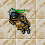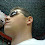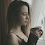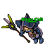## Wednesday, August 25, 2021

### TibiaGoals Riddles E11R06

Comment down below!

1.Tamarizz - Solidera
24!

Falcon coif = 4
Golden Boots = 1
(1 golden boot = 0.5)
Golden Helmet = 40

last equation:
4 + (40+0.5) = ?
4 + (20) = 24

2.Falcon coif = 4
Golden Helmet = 40
Golden boots = 1
Golden boot = 0.5

4 + 40 * 0.5 = 24

/Yeka

3.Hey! I hope you're having a great week so far. :)
I'll go with 24. :D
Thank you very much and have a wonderful Wednesday!

4.24

Shizurie

5.6.24

Xarkost Eilia

7.Falcon Coif = 4
Golden Boots = 1
Golden Helmet = 40

8.Falcon coif = 4
Golden helmet = 40
Golden boots = 1

40 x 0.5 = 20 + 4 = 24

Char: Dyaniixz

9.10.4+4+4=12
40+4+1=45
4-1=3
4+40*0,5=22... bebitha consentida

11.4+4+4=12
40+4+1=45
4-1=3
4+40*0,5=22... bebitha consentida

12.Tynusiiaa/Antica

Falcon coif - 4
Golden helmet - 40
golden boots - 1

13.14.15.16.17.4 + 40 x 0,5 = 24
Mian Stone'arrow

18.24

Skyelie Hasulhoff <3

19.24

Toxic Hasulhoff

20.24; Lord Neganek

21.22.the answer is 24 because boots represent a 1, falcon coif represents a 4 and the golden helmet represents a value of 40.

23.24.25.26.4+4+4=12
40+4+1=45
4-1=3
4+40*0,5=24.... Bebitha consentida

27.4+4+4=12
40+4+1=45
4-1=3
4+40*0,5=24.... Bebitha consentida

28.29.character name: pablo reformed

30.character name: pablo reformed

31.24
Eternal hunger
Antica

32.24
Eternal hunger
Antica

33.24
Eternal hunger
Antica

34.Respuesta

4+4+4=12
40+4+1=45
4-1=3

35.4 + 40 x 0.5 = 24
Character: Amy Meow

36.4+4+4=12
40+4+1=45
4-1=3
4+40*0=0.. Bebitha consentida

37.4+4+4=12
40+4+1=45
4-1=3
4+40*0=0... Bebitha consentida

38.Myo Disturbed

? = 24

39.40.24

Lovely olka

41.Congratulations to all participants! Correct answer is: 24.

Please remember to check your current score at Riddles / Information site!